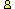CTK Exchange Front Page Movie shortcuts Personal info Awards Reciprocal links Terms of use Privacy Policy Cut The Knot! MSET99 Talk Games & Puzzles Arithmetic/Algebra Geometry Probability Eye Opener Analog Gadgets Inventor's Paradox Did you know?... Proofs Math as Language Things Impossible My Logo Math Poll Other Math sit's Guest book News sit's Recommend this siteCTK Exchange

 Subject: "Constucting a triangle instructions" Previous Topic | Next Topic
 ConferencesThe CTK ExchangeMiddle schoolTopic #132Printer-friendly copyEmail this topic to a friend Reading Topic #132
Gerald B.guest
May-20-08, 12:15 PM (EST)

"Constucting a triangle instructions"

 Dear Alex,I’ve been enjoying your math-focused website. I ran across this explanation for triangle construction given an angle “A”, an opposite side “a” and a height (at “A”) of “h”. I’m trying to follow the instructions but keep getting stuck at the first part. I just can’t figure out how to “Construct an arc (part of a circle) subtending segment BC = a such that for every point A on it, angle BAC equals the given angle A” How do I choose the radius of the arc or its starting point?Any hints?Insructions from the site: A, a, haConstruct an arc (part of a circle) subtending segment BC = a such that for every point A on it, angle BAC equals the given angle A. Draw a line parallel to BC at the distance ha. There could be 0,1, or 2 points of intersection of the line with the arc. In the latter case solutions are mirror images of each other; so even then there is at most one solution.

alexbCharter Member
2228 posts
May-20-08, 12:20 PM (EST)1. "RE: Constucting a triangle instructions"
In response to message #0

 Since angle A is given, you can draw a circle of an arbitrary radius R' and find a chord B'C' = a' that subtends the required angle. Now, you have to solve a proportionR/a = R'/a'for radius R.Gerlad B.guest
May-20-08, 01:31 PM (EST)

2. "RE: Constucting a triangle instructions"
In response to message #1

 OK I think I get it. Say angle A = 30 degrees. (Forget a and h for the moment). On a clock face, I find that if I put A’ at the centre, legs coming out at 30 degree angles cross the other edge of the clock one hour apart (say 6 and 7 o’clock). What I take from this is the radius of the clock and the linear distance between 6 and 7 (not in hours!). That gives me a ratio. Now, I am given the linear distance BC=a, so I can scale up my clock so that B’C’: BC = R’:RalexbCharter Member
2228 posts
May-20-08, 01:32 PM (EST)3. "RE: Constucting a triangle instructions"
In response to message #2

 That is almost correct. The central angle is twice the inscribed angle. So that if your A = 30 degrees, you may want to consider the arc between 6 and 8 o'clock instead of 6 and 7. The rest is OK.

 Conferences | Forums | Topics | Previous Topic | Next Topic
 Select another forum or conference Lobby The CTK Exchange (Conference)   |--Early math (Public)   |--Middle school (Public)   |--High school (Public)   |--College math (Public)   |--This and that (Public)   |--Guest book (Protected)   |--Thoughts and Suggestions (Public) Educational Press (Conference)   |--No Child Left Behind (Public)   |--Math Wars (Public)   |--Mathematics and general education (Public)You may be curious to have a look at the old CTK Exchange archive.